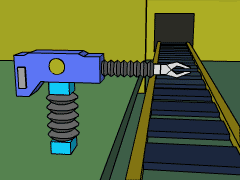Ch 1. Particle General Motion Multimedia Engineering Dynamics Position,Vel & Accel. Accel. varyw/ Time Accel. Constant Rect. Coordinates Norm/Tang. Coordinates Polar Coordinates RelativeMotion
 Chapter - Particle - 1. General Motion 2. Force & Accel. 3. Energy 4. Momentum - Rigid Body - 5. General Motion 6. Force & Accel. 7. Energy 8. Momentum 9. 3-D Motion 10. Vibrations Appendix Basic Math Units Basic Equations Sections Search eBooks Dynamics Fluids Math Mechanics Statics Thermodynamics Author(s): Kurt Gramoll ©Kurt GramollDYNAMICS - CASE STUDY IntroductionRobotic Arm Problem A robotic arm on an assembly line handles delicate components. To properly place these components, the position of the arm must be specified as a function of time. If, however, the acceleration of the arm is too great, the components may be damaged. What is known: The arm moves along a linear path. The position of the arm is given by x(t) = 0.3 t2 - 0.2 t3 m   for 0 ≤ t ≤ 1.5 seconds Questions Knowing the position of the arm as a function of time, What is the velocity as a function of time? What is the acceleration as a function of time? Approach Analyze the rectilinear motion of a particle to determine the relationship between position, velocity, and acceleration. Use differentiation to determine the velocity and acceleration of the arm.

Practice Homework and Test problems now available in the 'Eng Dynamics' mobile app
Includes over 400 problems with complete detailed solutions.
Available now at the Google Play Store and Apple App Store.